# 9. Some more examples.

Example 7: Determine the shortest curve y=y(x), y(0)=a, y(1)=b, that spans the area A together with the coordinate axes and the line x=1.Solution: The problem thus means that we should minimize the functional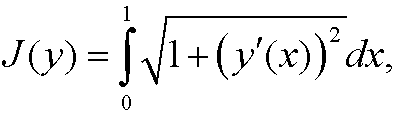under the constraint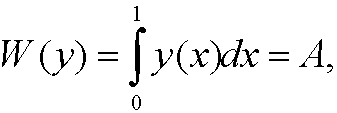where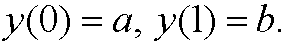By using the method from the previous part we must construct the functional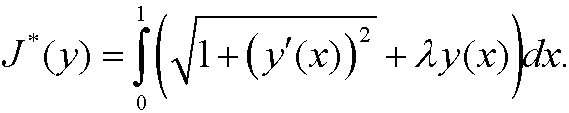A necessary condition for minimum is thatfulfills Euler’s equationIntegration now gives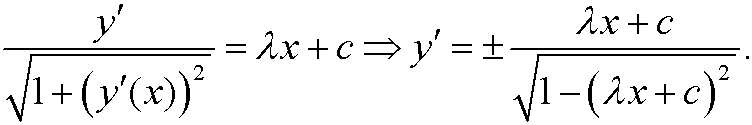If we integrate again we see that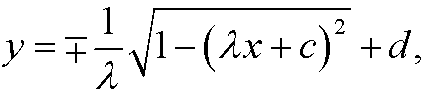that is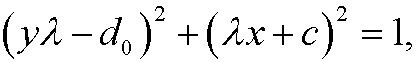which is a circle. The unknown constants, d0 and c are deterermined from the three conditionsExample 8:
(Chain line). A rope of length l and constant massper unit length hangs between two fixed points (a,y
1) and (b,y2) in the plane. What shape y(x) will the rope attain?Solution: A small element ds in the point (x,y) has the mass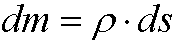and the potential energywith respect to y=0. The total potential energy is thusThe rope assumes a shape such that the potential energy is minimized. This means that we should minimize J(y) under the constraint of constant lengthWe thus constructand put it into the Euler equation. However, in this case L* does not explicitly depend on x and we can thus use Beltrami’s identitythat isIf we solve for y’ we getThis is a separable differential equation. Hence integration givesWe now make the change of variablesand then the left hand side integral transforms toWe multiply withand then take cosh(.) on both sides of the equality sign and use that it is an even function. We getIf we finally solve for y we getThis means that the shape of a hanging rop is a catenary (or chain line). The constants C, C2 andare determined by the boundary conditions and the curve length.

Example 9: Find the extremals to the triple integral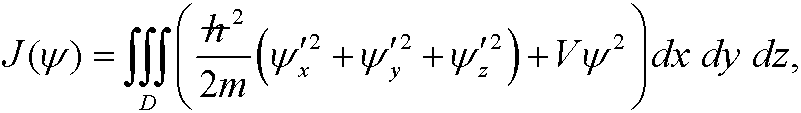under the constraintWe thus construct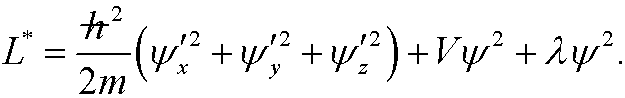The Euler equation in this case becomesthat isThis is the famous Schrödinger equation in quantum mechanics for a particle of mass m under the influence of a potential V. In this case D is R3and the constraints is the normalized probability condition for the wave function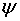, whose square is a probablility density (a measure of where a particle most likely will be). In general we can only find solutionsfor particular discrete values of the multiplier, identified as the possible energy levels that the particla may have.